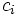# Class equation of a group

## Statement

Suppose$G$ is a finite group,$Z(G)$ is the center of$G$, and$c_1, c_2, \dots, c_r$ are all the conjugacy classes in$G$ comprising the elements outside the center. Let$g_i$ be an element in$c_i$ for each$1 \le i \le r$. Then, we have:$|G| = |Z(G)| + \sum_{i=1}^r |G:C_G(g_i)|$.

Note that this is a special case of the class equation of a group action where the group acts on itself by conjugation.

## Facts used

1. Class equation of a group action

## Proof

The proof follows directly from fact (1), and the following observations:

• When a group acts on itself by conjugation, the set of fixed points under the action is precisely the center of the group.
• The stabilizer of a point$g_i$ under the action by conjugation is precisely the centralizer of$g_i$.